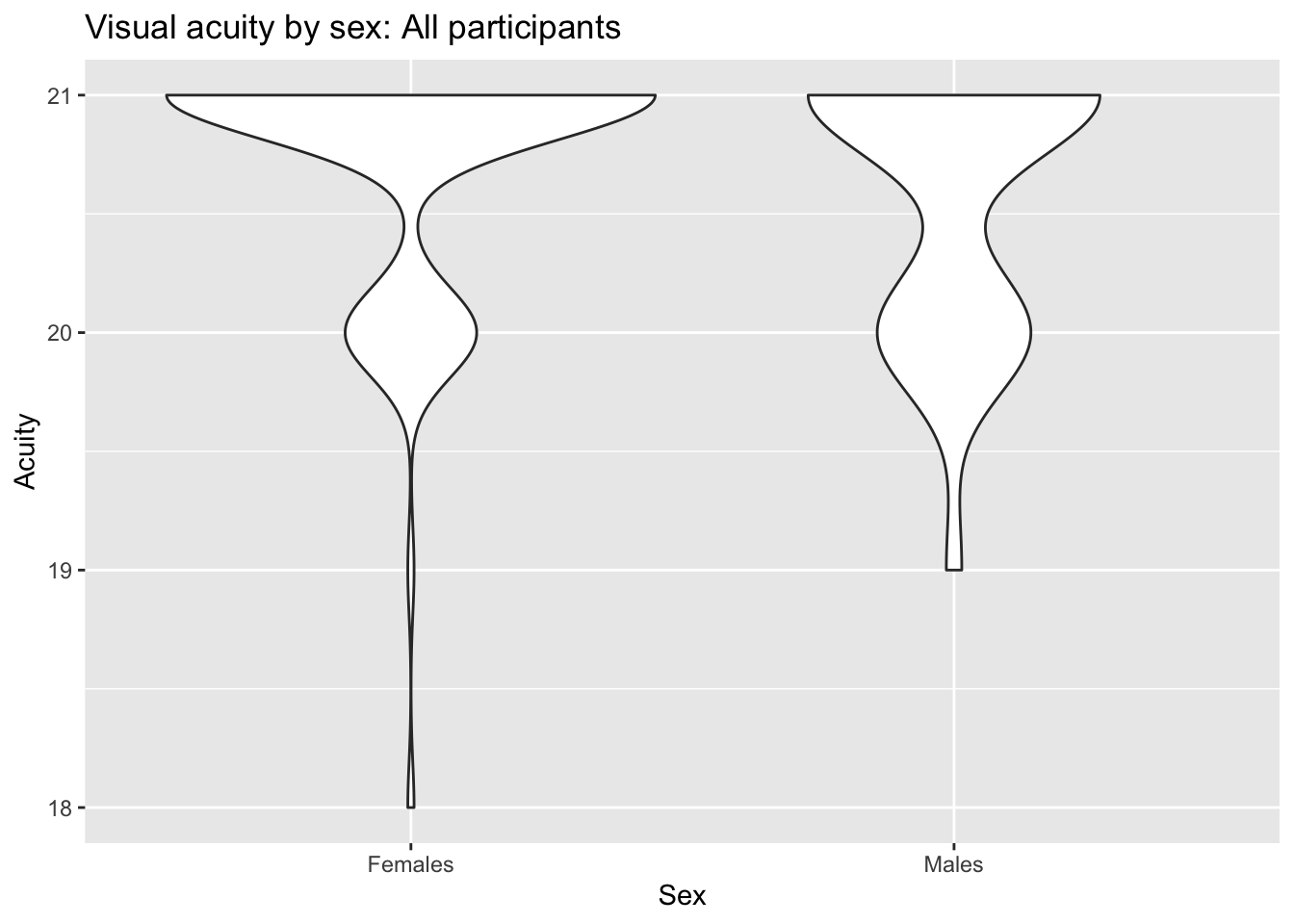# Purpose

This document shows our analyses following the preregistered plan. It also shows the results of trimming all cases whose scores on either of the visual perception measures exceed 2.5 SDs from the pooled mean.

# Analysis

## Import

We import data previously cleaned to remove cases where accuracy is lower than the guess rate and any zero scores in the survey measures.

``````# load the data and make each variable right
``````##
## ── Column specification ────────────────────────────────────────────────────────
## cols(
##   Participant = col_double(),
##   Sex = col_character(),
##   Race = col_character(),
##   Age = col_double(),
##   School_year = col_double(),
##   Major = col_character(),
##   Handedness = col_double(),
##   Glasses = col_double(),
##   Acuity = col_double(),
##   Color_vision = col_double(),
##   Stereo = col_double(),
##   Motion_duration_Threshold = col_double(),
##   Contrast_Sensitivity_Threshold = col_double(),
##   Vocabulary_scores = col_double(),
##   Vocabulary_scores_cor_num = col_double(),
##   Mental_Rotation_scores = col_double(),
##   Mental_Rotation_scores_both = col_double(),
##   Feminine_hobbies = col_double(),
##   Masculine_hobbies = col_double()
## )``````

## Rename variables

We rename some of the variables to make the exposition clearer.

``````df1 <- df1 %>%
dplyr::rename(., 'motion_thr' = 'Motion_duration_Threshold',
'contrast_thr' = 'Contrast_Sensitivity_Threshold',
'mental_rot' = 'Mental_Rotation_scores',
'mental_rot_both' = 'Mental_Rotation_scores_both',
'vocab' = 'Vocabulary_scores',
'vocab_num' = 'Vocabulary_scores_cor_num')``````

## Exclude outliers

Detect outliers three sd out of mean.

``````# define a function to remove outliers
FindOutliers <- function(data, sd_thresh = as.numeric(params\$outlier_sd_thresh)) {
sd = sd(data, na.rm = T)
mean = mean(data, na.rm = T)
# we identify extreme outliers
extreme.threshold.upper = (sd * sd_thresh) + mean
extreme.threshold.lower = -(sd * sd_thresh) + mean
result <-
which(data > extreme.threshold.upper |
data < extreme.threshold.lower)
print(result)
}

a <- lapply(df1, FindOutliers)``````
``## integer(0)``
``````## Warning in var(if (is.vector(x) || is.factor(x)) x else as.double(x), na.rm =
## na.rm): NAs introduced by coercion``````
``````## Warning in mean.default(data, na.rm = T): argument is not numeric or logical:
## returning NA``````
``## integer(0)``
``````## Warning in var(if (is.vector(x) || is.factor(x)) x else as.double(x), na.rm =
## na.rm): NAs introduced by coercion

## Warning in var(if (is.vector(x) || is.factor(x)) x else as.double(x), na.rm =
## na.rm): argument is not numeric or logical: returning NA``````
``````## integer(0)
##   1 15 21 56 61 85
##   15  21  34  85  97 104``````
``````## Warning in var(if (is.vector(x) || is.factor(x)) x else as.double(x), na.rm =
## na.rm): NAs introduced by coercion

## Warning in var(if (is.vector(x) || is.factor(x)) x else as.double(x), na.rm =
## na.rm): argument is not numeric or logical: returning NA``````
``````## integer(0)
##     1  21  29  45  47  59  61  66 100 125
## integer(0)
##   39  59 104
##  82 92
##    2  42  47  74  79 104
##  125 128
##    4  16  49  77  99 129
##  110
##  48
## integer(0)
## integer(0)
##  39 58
##  20 33``````
``a``
``````## \$Participant
## integer(0)
##
## \$Sex
## integer(0)
##
## \$Race
## integer(0)
##
## \$Age
##   1 15 21 56 61 85
##
## \$School_year
##   15  21  34  85  97 104
##
## \$Major
## integer(0)
##
## \$Handedness
##     1  21  29  45  47  59  61  66 100 125
##
## \$Glasses
## integer(0)
##
## \$Acuity
##   39  59 104
##
## \$Color_vision
##  82 92
##
## \$Stereo
##    2  42  47  74  79 104
##
## \$Motion_duration_Threshold
##  125 128
##
## \$Contrast_Sensitivity_Threshold
##    4  16  49  77  99 129
##
## \$Vocabulary_scores
##  110
##
## \$Vocabulary_scores_cor_num
##  48
##
## \$Mental_Rotation_scores
## integer(0)
##
## \$Mental_Rotation_scores_both
## integer(0)
##
## \$Feminine_hobbies
##  39 58
##
## \$Masculine_hobbies
##  20 33``````

Question: Do we care about outliers in `Age`, `Color_vision`, or `Stereo` vision?

ROG Answer: Probably not. We might care about outliers in visual acuity, though.

So, let’s do some quick plots to see what’s going on.

``````df1 %>%
ggplot(.) +
aes(y = Acuity, x = Sex) +
geom_violin() +
ggtitle('Visual acuity by sex: All participants')``````
``## Warning: Removed 1 rows containing non-finite values (stat_ydensity).````````df1[-a[['Acuity']],] %>%
ggplot(.) +
aes(y = Acuity, x = Sex) +
geom_violin() +
ggtitle('Visual acuity by sex: Outliers excluded')``````
``## Warning: Removed 1 rows containing non-finite values (stat_ydensity).``